# PTA作业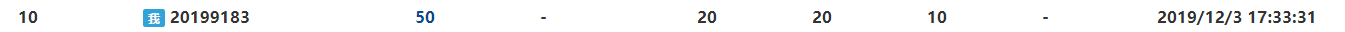# 1.PTA实验作业

1.1题目名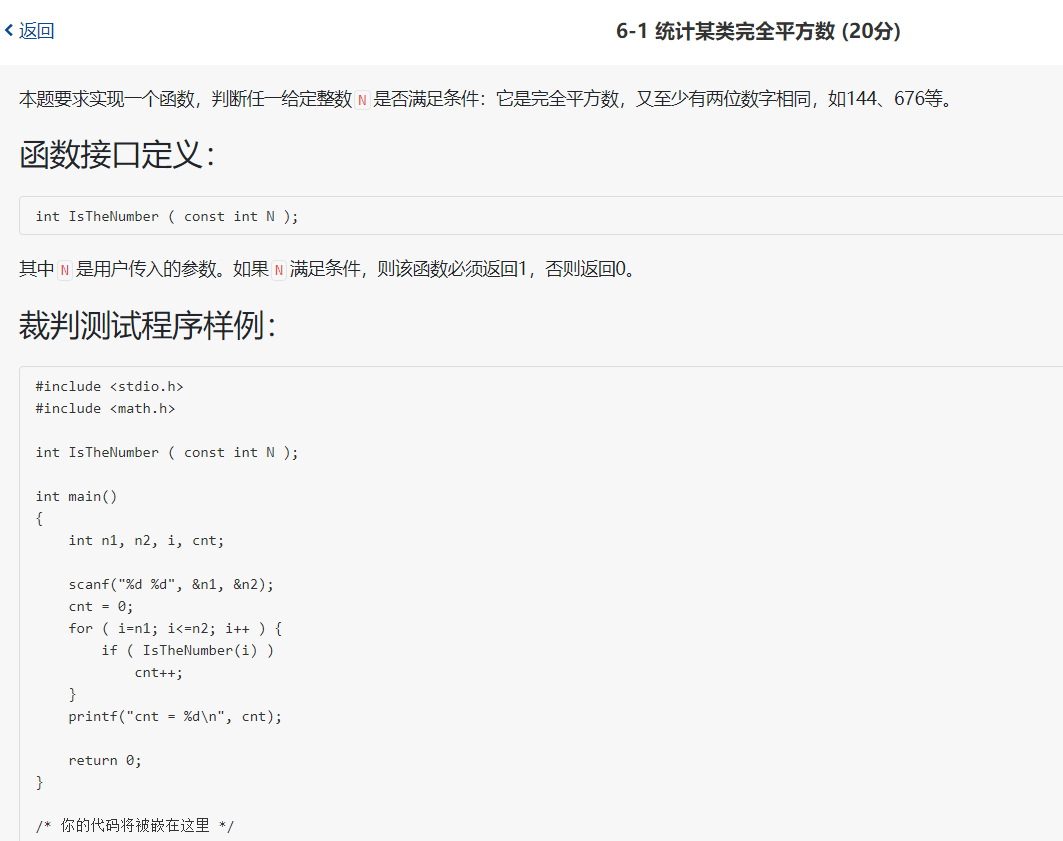1.1.1数据处理

while(a!=0){
for(i=0;i<=9;i++){
if(a%10i){
num[i]+=1;
if(num[i]
2)
return 1;}}
a=a/10;
1.1.2实验代码截图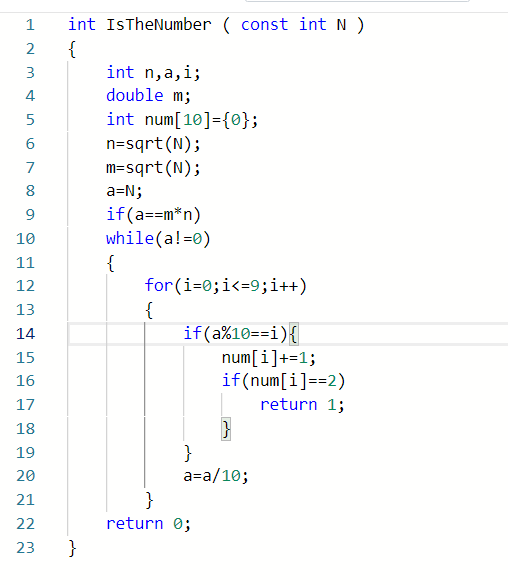1.1.3造测试数据

105 500 | cnt = 6 | 测试是否与题目一致
0 10 | cnt = 1 | 随机输入的数据进行测试

1.1.4 PTA提交列表及说明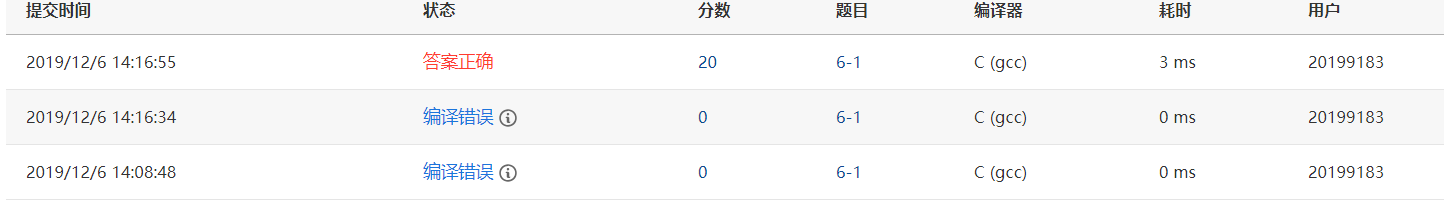1.2题目名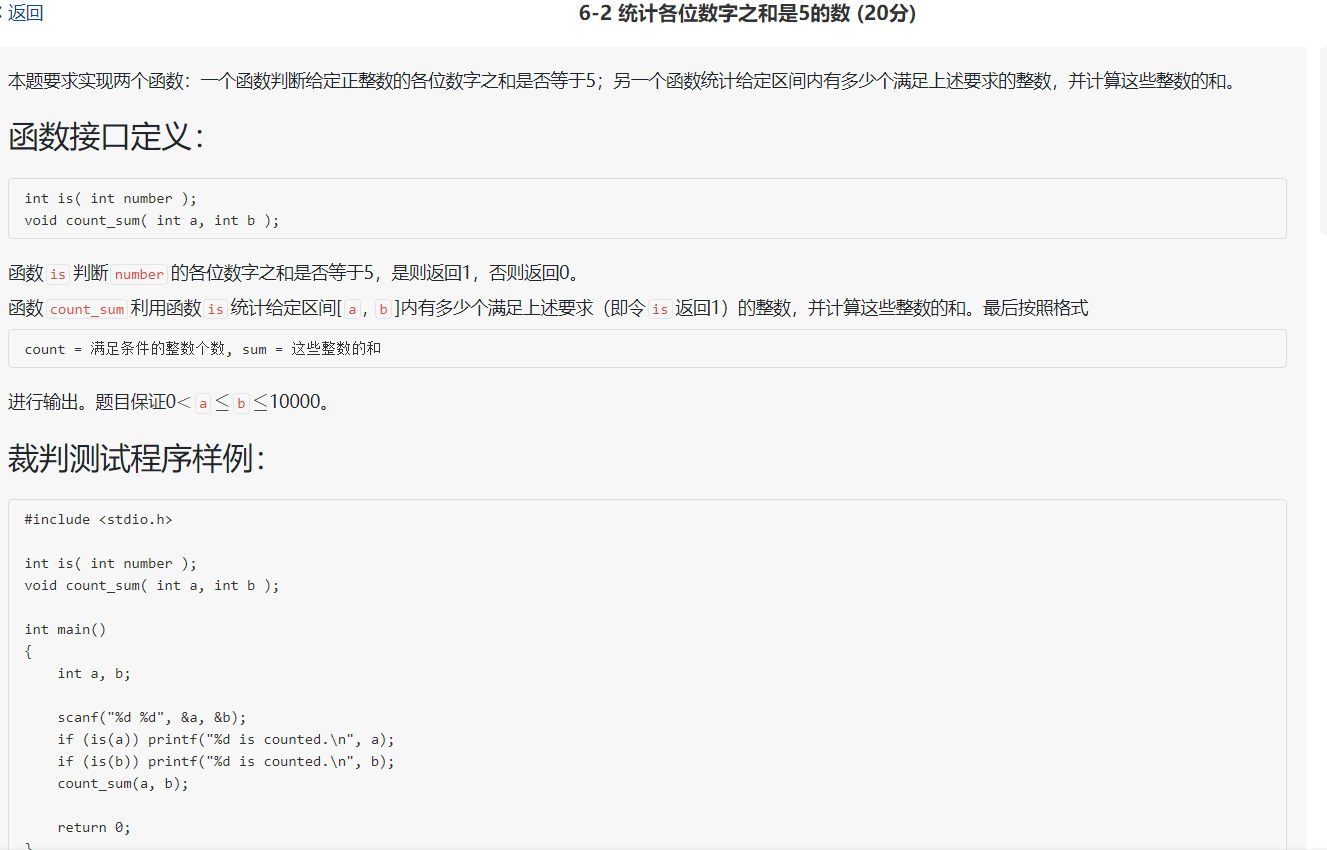int s=0,t=0;
while(n!=0){
t=n%10;
n/=10;
s+=t;
}
if(s==5)
return 1;
else
return 0;

int sum=0,count=0,i;
for(i=a;i<=b;i++){
if(is(i)){
count++;
sum+=i;

1.2.2实验代码截图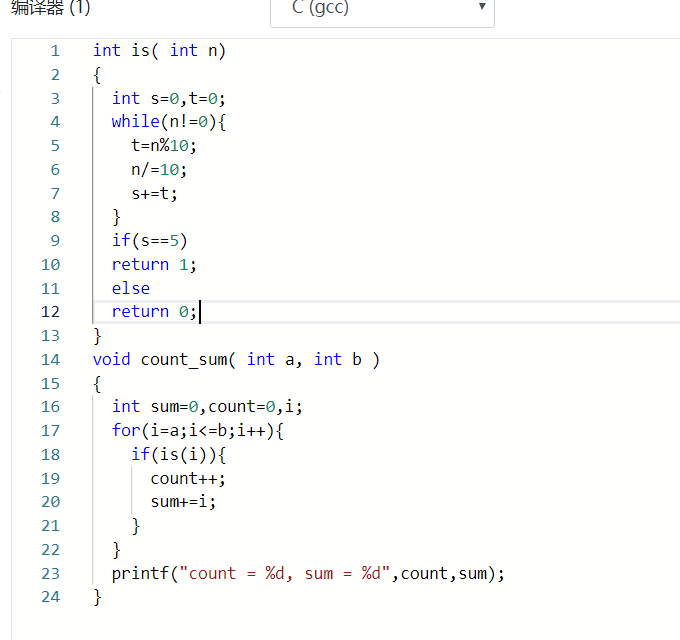104 999 104 is counted. 随机输入数据进行测试
1000 1999 count = 15, sum = 17220 随机输入较大的数据进行测验

1.2.4 PTA提交列表及说明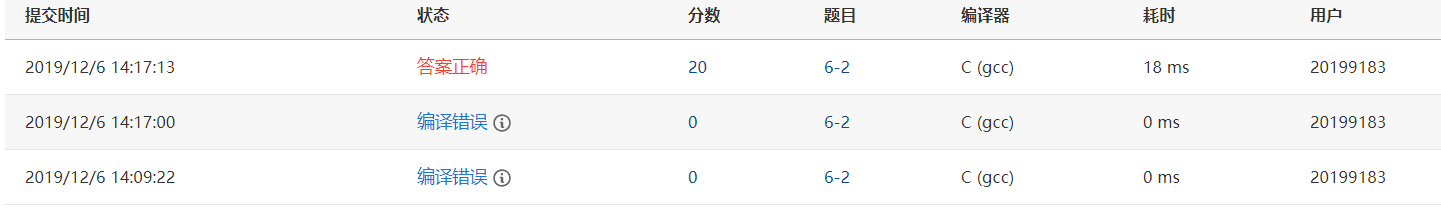# 2.代码互评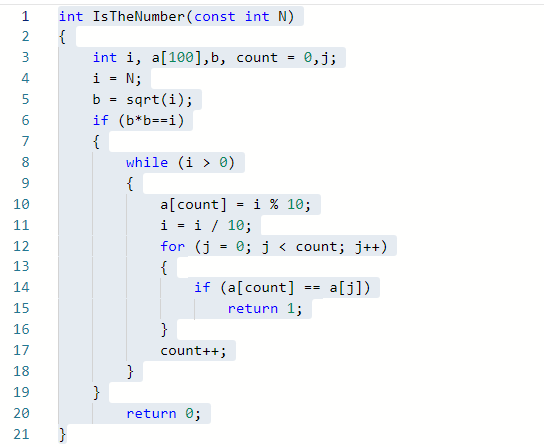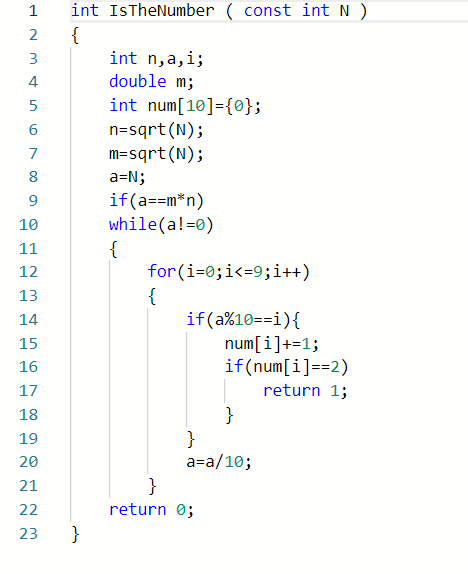3.1 学习进度条

3.2累计代码行和博客字数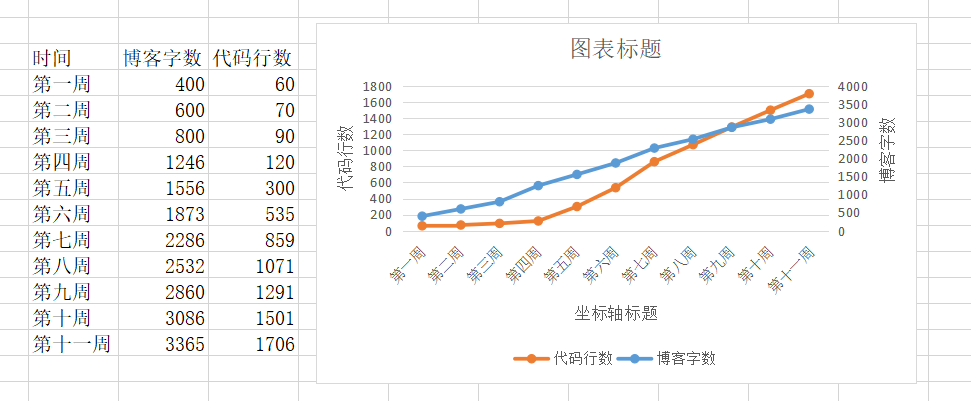3.3学习感悟

# 思维导图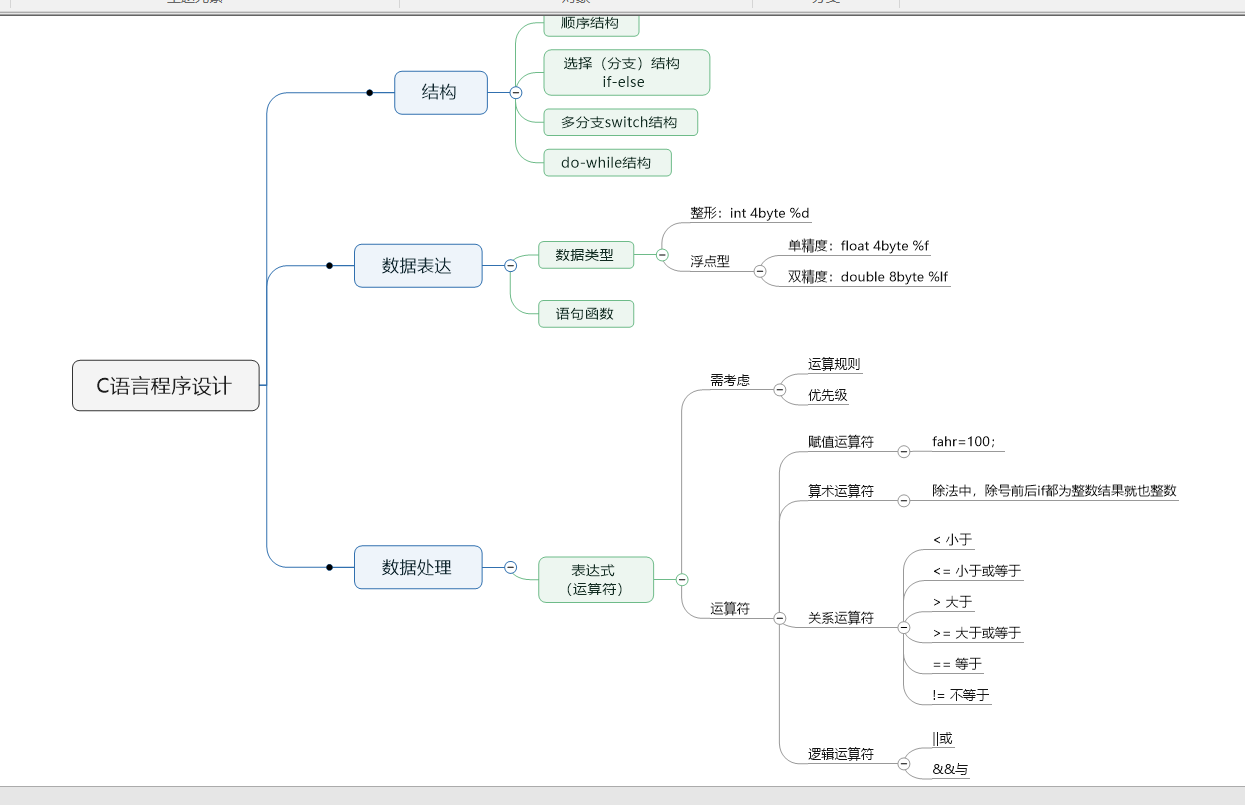posted @ 2019-12-06 14:47  Suless  阅读(80)  评论(1编辑  收藏